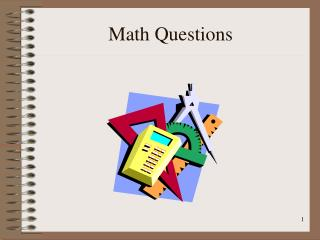DownloadDownload PresentationMath Questions

Math Questions

Download PresentationMath Questions

- - - - - - - - - - - - - - - - - - - - - - - - - - - E N D - - - - - - - - - - - - - - - - - - - - - - - - - - -
Presentation Transcript

1. Math Questions

2. Math Questions • Length x Width x Water Depth = cubic feet

3. Math Questions • Length x Width x Water Depth = cubic feet 7.5 gallons per cubic foot

4. Math Questions • Length x Width x Water Depth = cubic feet 7.5 gallons per cubic foot 10 x 5 x 5 = 250 cubic feet

5. Math Questions • Length x Width x Water Depth = cubic feet 7.5 gallons per cubic foot 10 x 5 x 5 = 250 cubic feet 250 x 7.5 = 1875 gallons

6. Math Questions • 1500 gallon tank

7. Math Questions • 1500 gallon tank • It has 1250 gallons in it.

8. Math Questions • 1500 gallon tank • It has 1250 gallons in it. • Your pump does 50 gallons per minute (gpm)

9. Math Questions • 1500 gallon tank • It has 1250 gallons in it. • Your pump does 50 gallons per minute (gpm) • How many minutes to empty the tank?

10. Math Questions • 1500 gallon tank • It has 1250 gallons in it. • Your pump does 50 gallons per minute (gpm) • How many minutes to empty tank? 1250 50 = 25minutes